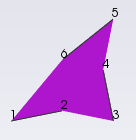# Hide internal edges of quadratic cells

Hi!
In my application I am trying to add some quadratic cell (vtkQuadraticQuad and vtkQuadraticTriangle) in a vtkUnstructuredGrid. The problem is that, as in the following example (https://lorensen.github.io/VTKExamples/site/Python/GeometricObjects/IsoparametricCellsDemo/) there are some internal edges that are displayed. Therefore, it is hard to see if displayed elements are quadratic ones or simply several triangles…
In paraview I managed to create a quadratic quad and internal edges seems not to be displayed I assume then that it may be possible to do the same under vtk.
Does anybody have an idea of how to do that?

Hi all!
Sorry to repost but my problem is always there and I found no solution… Nobody have any idea on how to remove internal edges of quadratic elements?
Maybe it can be possible by removing edges of cells actor (EdgeVisibilityOff) and adding edges separatly in another actor with a vtkExtractEdges filter. But this seems to much complicated for such a simple thing…

Hi,

You can hide edges by setting a vtkUnsignedCharArray named `vtkEdgeFlags` as point data. This array is read at rendering and edges flagged as ‘0’ are not drawn. Points are mapped to edges counter clockwise if I remember correctly, but you will figure this out pretty fast by playing with it.

If you want an example of its use, look at class `vtkHyperTreeGridGeometry` (in VTK master), and don’t hesitate to ask questions if you run into issues going forward.

Sound promising after activating GetPointData().SetActiveAttribute(vtkEdgeFlagsArray.GetName(), vtk.vtkDataSetAttributes.EDGEFLAG) but I’m afraid this doesn’t works.
For example, if I take a quadratic triangle with end points 1, 3 and 5 and middle points 2, 4 and 6.
If I turn edgeflag to 0 for points 2, 4 and 6 it hides internal edges but also edges between points 2 and 3, 4 and 5 and 6 and 1. In fact in a quadratic triangle there is 9 edges including internals and only 6 points at all. So how can we choose to hide only the 3 internal edges?I’m assuming that you are dealing with triangles right? If so, you would need to duplicate the points and attach to the points of the cells inside the hull zero vtkEdgeFlags. This is how it is handled in `vtkHyperTreeGeometry`.

Let me reformulate. For any point that needs to have an erased edge, you should associate a point whose `vtkEdgeFlags` is `0` in addition to a point whose `vtkEdgeFlags` is `1`, and connect the triangles accordingly. If you need to erase multiple edges originated from one point, you can link them to the same point with flag `0`.

In the vtkEdgeFlags example they draw a square using 4 different triangles. In my case it means that I should create several triangle cells to represent one quadratic element and this is a main change in my code.

By looking around, someone pointed me to this old test file that seems to generate what you intend to do: VisualizeLagrangeTriangleMesh.cc (9.4 KB)

It might be `vtkDataSetSurfaceFilter` which does the trick, but I didn’t test enough to figure out exactly where the magic happens.

Here is a screen of the result rendered in ParaView:

I hope you figure out how to apply this example to your setup soon!

For hiding the internal edge of quadratic cells in VTK you could use vtkUnstructuredGridGeometryFilter and vtkExtractEdges
C# sample code:

``````        vtkUnstructuredGridGeometryFilter uggFilter = vtkUnstructuredGridGeometryFilter.New();
uggFilter.SetInput(uGrid);
vtkExtractEdges edges = vtkExtractEdges.New();
edges.SetInputConnection(uggFilter.GetOutputPort());
vtkDataSetMapper edgeMapper = vtkDataSetMapper.New();
edgeMapper.SetInputConnection(edges.GetOutputPort());

vtkActor EdgeActor = vtkActor.New();
EdgeActor .SetMapper(edgeMapper);
EdgeActor .GetProperty().SetEdgeVisibility(1);
EdgeActor .GetProperty().SetEdgeColor(0,0.5,0);